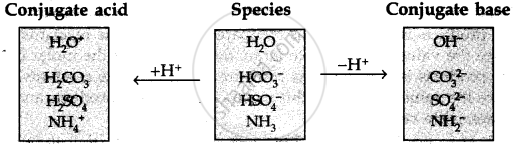CBSE (Science) Class 11CBSE
Share

# The Species: H2o,Hco−3hco3-,Hso−4hso4- and Nh3 Can Act Both as BröNsted Acids and Bases. for Each Case Give the Corresponding Conjugate Acid and Base - CBSE (Science) Class 11 - Chemistry

ConceptConcept of Acids, Bases and Salts

#### Question

The species: H2O,HCO_3^(-),HSO_4^(-) and NH3 can act both as Brönsted acids and bases. For each case give the corresponding conjugate acid and base.

#### Solution 1

The table below lists the conjugate acids and conjugate bases for the given species.

 Species Conjugate acid Conjugate base H_2O H_3O^(+) OH^(-) HCO_3^(-) H_2CO_3 CO_3^(2-) HSO_4^(-) H_2SO_4 SO_4^(2-) NH_3 NH_4^(+) NH_2^(-)

#### Solution 2Is there an error in this question or solution?

#### APPEARS IN

Solution The Species: H2o,Hco−3hco3-,Hso−4hso4- and Nh3 Can Act Both as BröNsted Acids and Bases. for Each Case Give the Corresponding Conjugate Acid and Base Concept: Concept of Acids, Bases and Salts.
S